# IMO Class 3 level 2 Question Paper - Set 6

Check these Class 3 IMO Level 2 questions from previous years Maths Olympiad exams.

For our paid members, we offer comprehensive benefits, including access to detailed explanations for all questions in our Chapterwise Test Series and Mock Test Series. Our paid members have the opportunity to attempt more than a dozen tests for each chapter covered in the SOF IMO syllabus for Class 3. They can attempt each test multiple times to enhance their learning experience.Our courses can be bought at our BUY COURSES section.

Our Mock Tests for Class 3 IMO follow the exact pattern of SOF IMO in terms of questions, marking and time alloted for completion. This helps the students to experience of the real time exam environment and assess their exam preparedness. Since we follow the same marking system , the scores obtained at the end of each test will help identify students' weaknesses and assist them in improving.

Olympiadtester has displayed a few Chapterwise tests and Mock tests in the FREE SECTION.

1) The area of rectangle below is 75 cm2. Find the perimeter of the rectangle.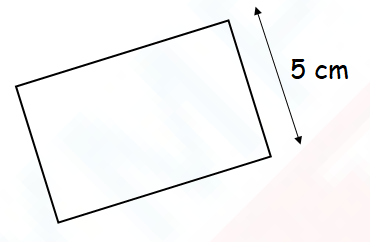OPTIONS

1. 15 cm
2. 20 cm
3. 40 cm
4. 80 cm

2) The figure below is made up of 1-metre squares. Find the perimeter of the figure.OPTIONS

1.  24 m
2. 16 m
3. 9 m
4. 8 m

3) The figure below is made up of 5 identical rectangles. Find the perimeter of the figure.OPTIONS

1. 160 cm
2. 320 cm
3. 600 cm
4. 800 cm

4) How many right angles are there inside the figure below?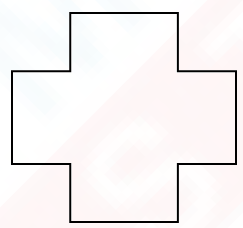OPTIONS

1. 16
2. 12
3. 8
4. 4

5) The area of each square is 4m2. What is the total area of the figure below?OPTIONS

1. 52 m2
2. 48 m2
3. 44 m2
4. 12 m2

6) The figure below is not drawn to scale. Find the perimeter of the figure.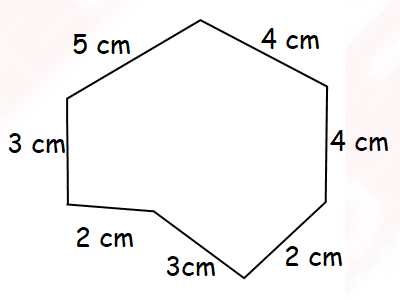OPTIONS

1. 28 cm
2. 25 cm
3. 23 cm
4. 22 cm

7 The figure below shows the layout of Arya's garden. Arya wants to fence the 2 rectangulat flower beds in her garden. ​​​​​​​How much fencing will she require in all?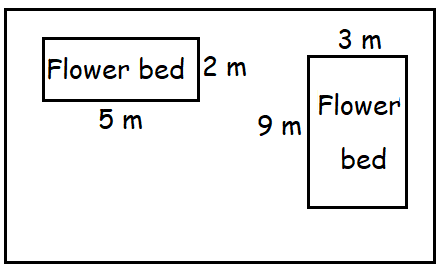OPTIONS

1. 26 m
2. 29 m
3. 38 m
4. 56 m

8) Find the area of the shaded figure.OPTIONS

1. 16 cm2
2. 18 cm2
3. 20 cm2
4. 22 cm2

9) The figure below is not drawn to scale. Find the perimeter of the figure.OPTIONS

1. 26 cm
2. 35 cm
3. 42 cm
4. 56 cm

10) Which one of the following angles is less than 90°?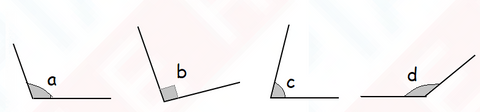OPTIONS

1. Angle a
2. Angle b
3. Angle c
4. Angle d

11) Which one of the following angles is the biggest?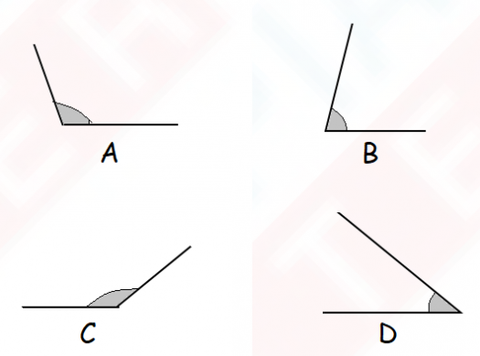12) The figure below is made up of 8 equal sides. The total length of 3 of its sides is 84 m. What is the perimeter of the figure?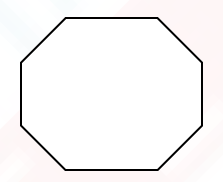13) How many triangle As are needed to form the figure below?14) Study the figure below.
​​How many angles in the figure are smaller than a right angle?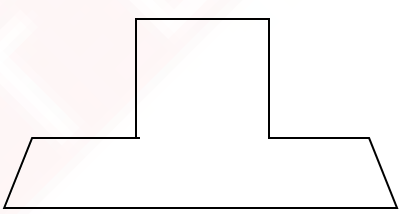15) Two sisters are sewing a rectangular quilt with square pieces of unused cloth. The area of the quilt is 225 m2. The side of each square piece of unused cloth is 3 m. How many square pieces of cloth do they need for the quilt?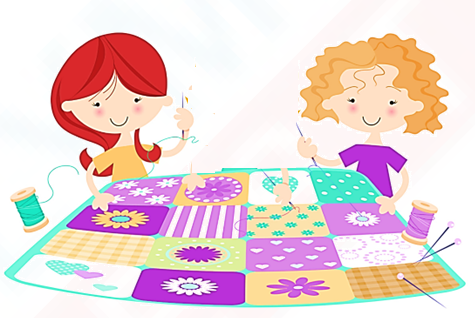Olympiadtester has displayed a few Chapterwise tests and Mock tests in the FREE SECTION.